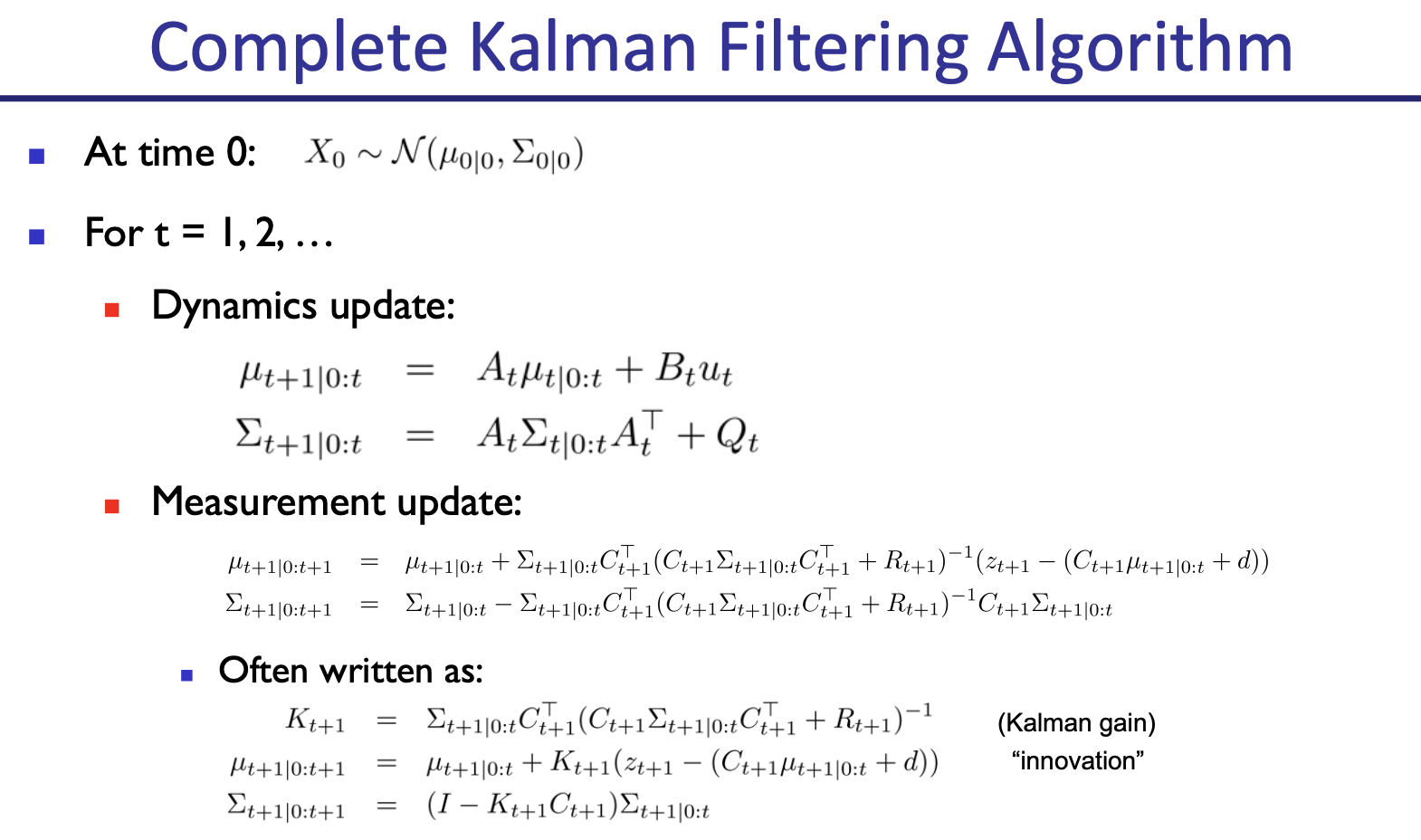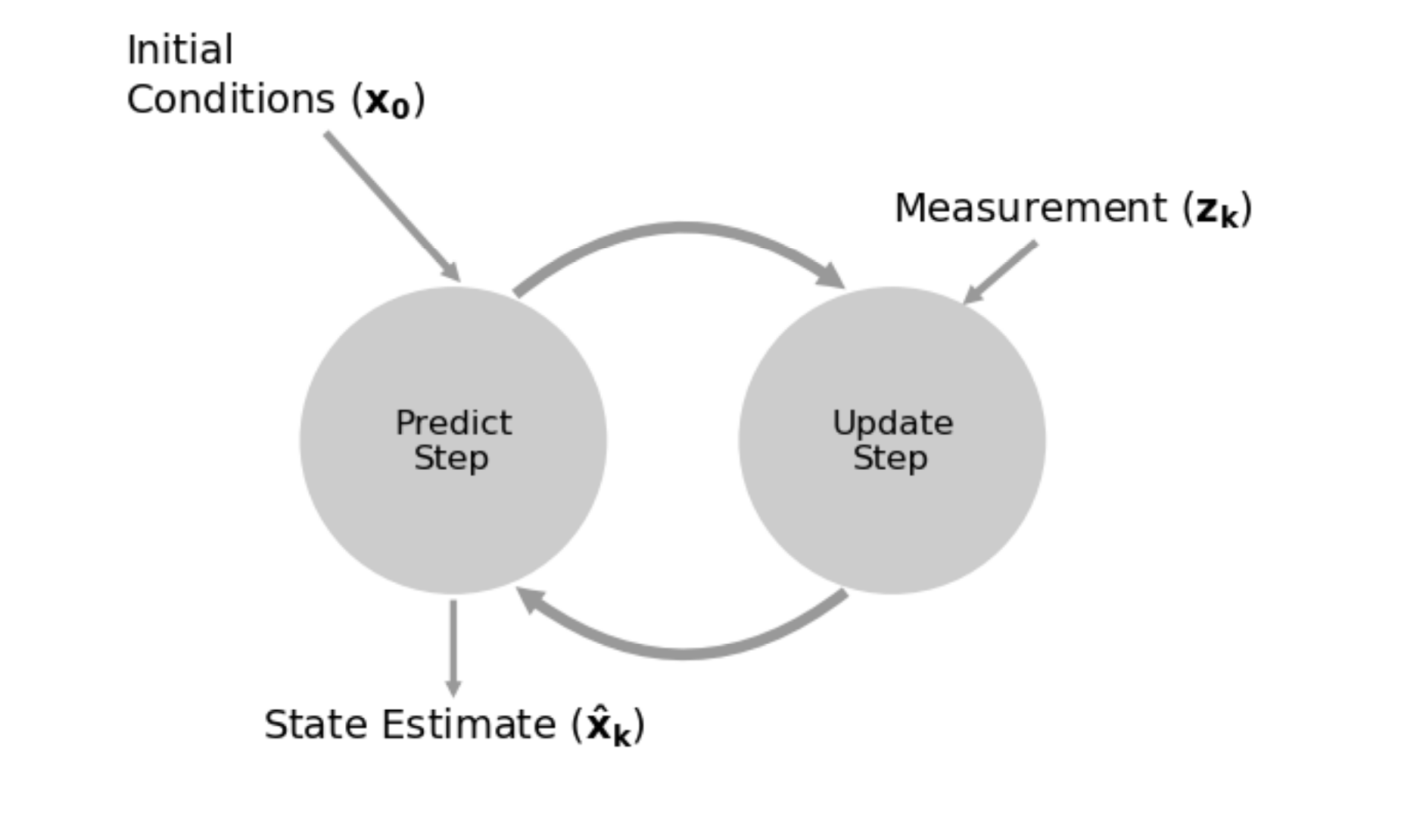State Estimation

# Kalman Filter §

A Kalman filter is a mathematical algorithm for State Estimation based on noisy measurements.

• Recursive algorithm that uses a combination of predictions and measurements to estimate the state of a system over time

2 steps:

1. Prediction
• uses the system model to predict the state of the system based on the previous state estimate and the control inputs to the system
2. Update
• uses the current measurement to correct the previous state estimate, and produce a new, more accurate state estimate

I was learning about Kalman Filters back in like Grade 9 when I was trying to build a self-balancing rocket. I watched through quite a few lectures.

i was trying to build a Rocket.

They used those things in rockets! Actually, Kalman filters are used everywhere. Self-driving cars, drones, everything I am interested in actually uses the Kalman filter.

This is the repository:

From CS287, a Kalman filter is just a special case of Bayes Filter, where dynamics and sensory models linear Gaussians.### Notes from the book §

If we only form estimates from the measurement then the prediction will not affect the result. If we only form estimates from the prediction then the measurement will be ignored. If this is to work we need to take some kind of blend of the prediction and measurement.

“Multiple data points are more accurate than one data point, so throw nothing away no matter how inaccurate it is”